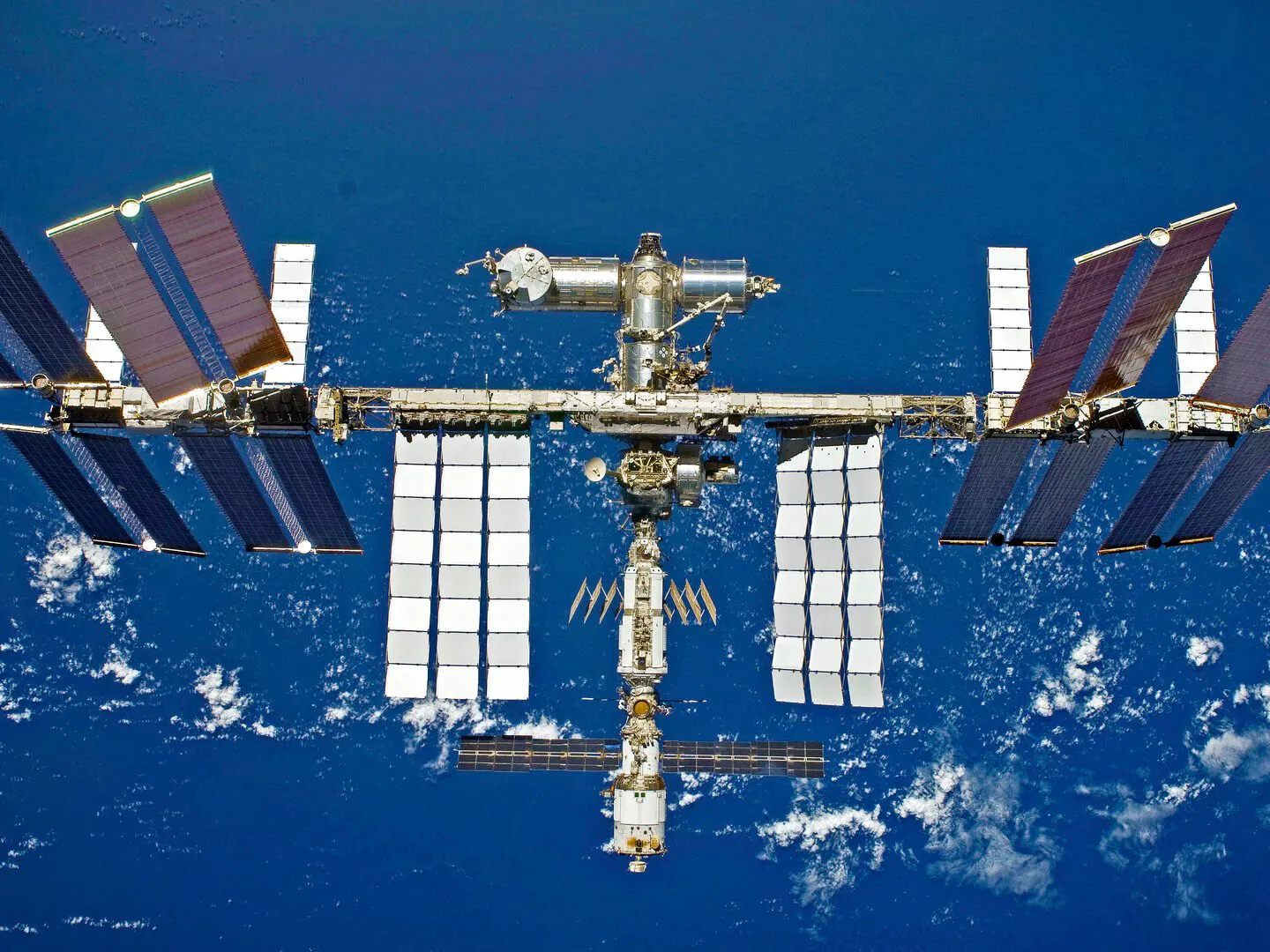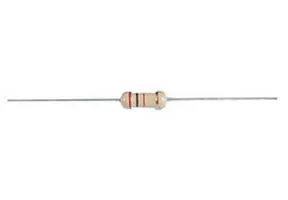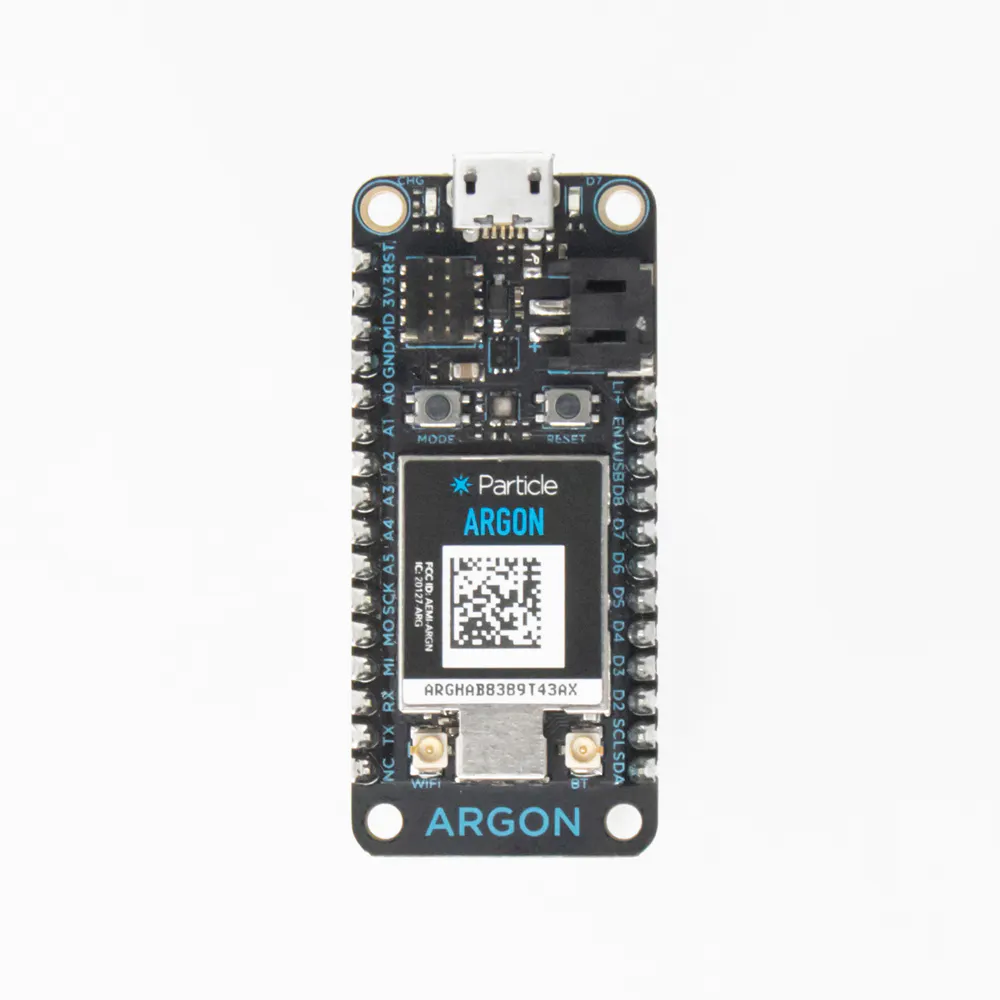Published

# Lane Tech HS -- PCL -- ISS Tracker

The International Space Station completes one Earth orbit every 90 minutes. Where is it now?

IntermediateFull instructions provided10 hours510## Things used in this project

### Hardware components

×72Through Hole Resistor, 560 ohm
×1Particle Argon
×1

### Software apps and online servicesParticle Build Web IDE
 Open Notify

### Hand tools and fabrication machinesSoldering iron (generic)

## Code

### Visualizing ISS Orbit Code

C/C++
```// This #include statement was automatically added by the Particle IDE.
#include <ArduinoJson.h>

StaticJsonDocument<200> doc;

// This #include statement was automatically added by the Particle IDE.
#include <neopixel.h>

SYSTEM_MODE(AUTOMATIC);

//set LED grid size
const int LEDlength = 12;
const int LEDup = 6;

// IMPORTANT: Set pixel COUNT, PIN and TYPE
const int PIXEL_COUNT = LEDup * LEDlength;
#define PIXEL_PIN D4
#define PIXEL_TYPE WS2812B

void setup() {
Particle.subscribe("hook-response/ISSloc", myHandler, MY_DEVICES);
Serial.begin(9600);

strip.begin();
strip.show();
}

void loop() {
// Get some data
String data = String(10);
// Trigger the integration
Particle.publish("ISSloc", data, PRIVATE);
// Wait 5 seconds
delay(5000);
}

void myHandler(const char *event, const char *data)
{
//reset the LEDs that are on
for(uint16_t i=0; i<strip.numPixels(); i++) {
strip.setPixelColor(i, strip.Color(0, 0, 0));
}

const char* json = data;
deserializeJson(doc, json);

//retrieve the latitude and longitude from the JSON object
float lat = doc["iss_position"]["latitude"];
float lon = doc["iss_position"]["longitude"];
Serial.println(lat);
Serial.println(lon);

//convert the lat and lon into LED grid coordinates
int Lat = (int) lat;
int Lon = (int) lon;
int longitude = map(Lon, -180, 180, 1, (LEDlength + 1));
int latitude = map(Lat, 90, -90, 1, (LEDup + 1));
//in order to get the coordinates split into the right number of zones,
//the number of zones in the LED grid needs to be increased by 1
//the map function only maps to the highest map to number when
//the highest map from number is achieved

//manage the cases where the latitude = -90 or longitude = 180
if (longitude == (LEDlength + 1)){
longitude = LEDlength;
}
if (longitude == (LEDup + 1)){
longitude = LEDup;
}

Serial.println(latitude);
Serial.println(longitude);

//latitude is not needed to map just the longitude (when LED = 1)
if(LEDup == 1){
strip.setPixelColor(longitude, strip.Color(50,50,50));
strip.show();
}

//convert the coordinates to an LED number on the grid
else{
int LEDnum;
LEDnum = (LEDlength*(latitude - 1) + longitude);
Serial.print(LEDnum);
strip.setPixelColor((LEDnum-1), strip.Color(50,50,50));
strip.show();
}
}
```

## Credits

### Sydney Curran

3 projects • 2 followers